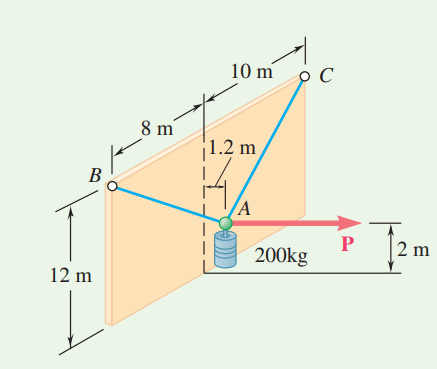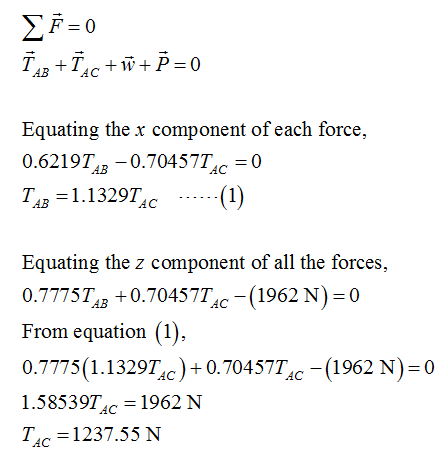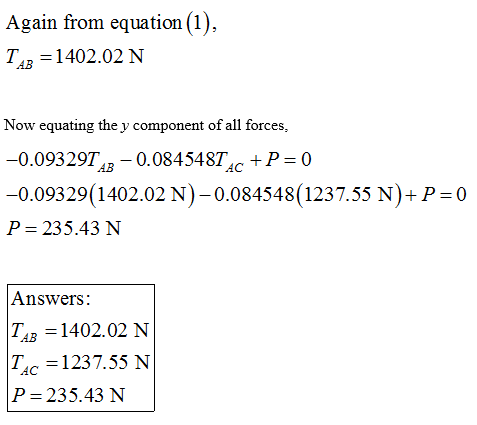# A 200-kg cylinder is hung by means of two cables AB and AC that are attached to the top of a vertical wall. A horizontal force P perpendicular to the wall holds the cylinder in the position shown. Determine the magnitude of P and the tension in each cable.

Question-AnswerCategory: Engineering MechanicsA 200-kg cylinder is hung by means of two cables AB and AC that are attached to the top of a vertical wall. A horizontal force P perpendicular to the wall holds the cylinder in the position shown. Determine the magnitude of P and the tension in each cable.

### A 200-kg cylinder is hung by means of two cables AB and AC that are attached to the top of a vertical wall. A horizontal force P perpendicular to the wall holds the cylinder in the position shown. Determine the magnitude of P and the tension in each cable.Consider the diagram shown below for the given system.Here, TAB and TAC are the tension in the strings AB and AC respectively.
The position vector for the AB is,The position vector for AC is,Step 2

Tensions in the strings in the vector form can be represented as,Step 3

From the equilibrium of forces,